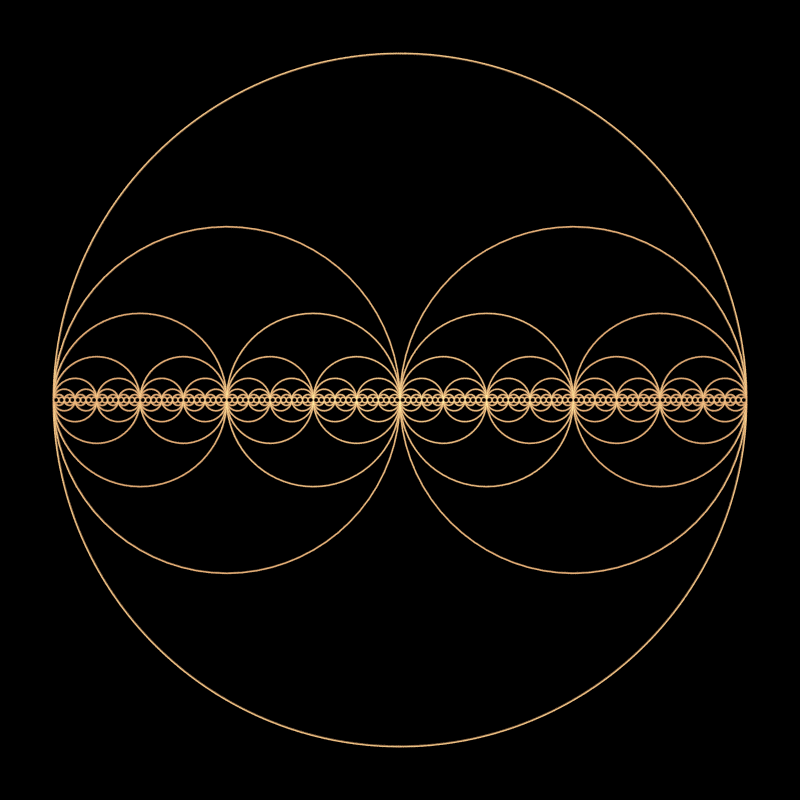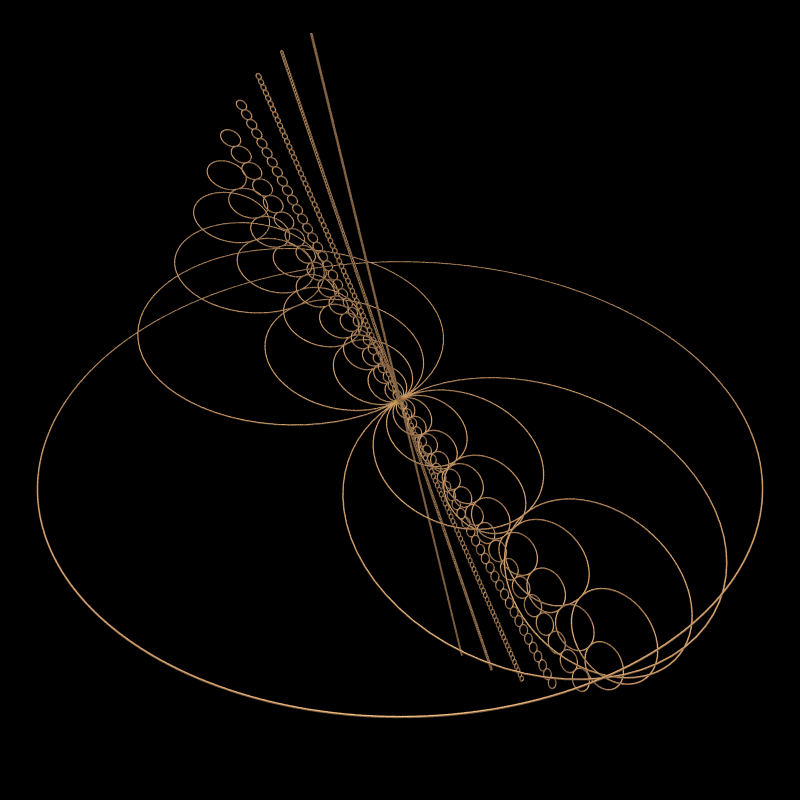# Circular Infinity

Written by Paul Bourke

August 2003

Based upon work (and named) by Eep2

The "trivially" self similar object shown here bears a resemblance to many other forms including in particular the Cantor dust set. On each iteration each circle is filled with two circles of half the radius, these two circles are mutually tangential to each other as well to the circle they are within. As such this form seems related to the Apollony fractal except we now need an extra constraint, namely that the filling circles are align on one axis.The above image was created using a very simple PovRay macro as given below.

```// Define the macro that creates a single circle
// SCx and SCy is the center
// SCr is the radius
// SCd is the angle resolution in degrees, should be an integer divisor of 360
#macro SingleCircle(SCx,SCy,SCr,SCd)
#declare i = 0;
#while (i <= 360)
#declare xnext = SCx+SCr*cos(radians(i));
#declare ynext = SCy+SCr*sin(radians(i));
#if (i < 360)
sphere { <xnext,ynext,0>, loopthickness texture { looptexture } }
#end
#if (i > 0)
cylinder { <xlast,ylast,0>, <xnext,ynext,0>, loopthickness texture { looptexture } }
#end
#declare xlast = xnext;
#declare ylast = ynext;
#declare i = i + SCd;
#end // while
#end // macro
```

This might be called within a PovRay model file as follows, indeed this is exactly the version used to create the above image to 8 levels. Note the main magic below is getting the spacing correct for each iteration depth.

```#declare loopthickness = 0.003;
#declare loopradius = 1;
union { SingleCircle(0,0,loopradius,1) } // Outer circle

#declare nn = 1;
#declare iterations = 1;
#while (iterations < 8)
#declare loopradius = loopradius / 2; // Radius is halved at each iteration
#declare nn = 2 * nn;
#declare n = -(nn - 1);
#declare resol = 10; // Create smaller circles more coarsely
#if (iterations < 3) #declare resol = 2; #end
#if (iterations < 5) #declare resol = 5; #end
#while (n <= nn - 1)
union { SingleCircle(-n/nn,0,loopradius,resol) }
#declare n = n + 2;
#end
#declare iterations = iterations + 1;
#end // iterations
```

Since this is now a 3D model we can obviously look at from different angles and do various other transformations which can of course be animated.